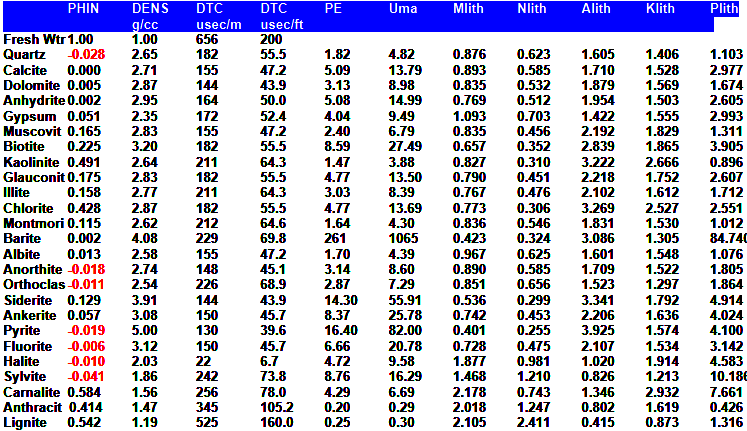Porosity from Lithology Models
This method assumes that lithology is known from a UMA - DENSMA 3 mineral model or some other method that will determine mineral volumes accurately. The method can also be used if Vmin1 and Vmin2 (and Vmin3 if desired) are derived from sonic density neutron (Mlith/Nlith or DELTMA/DENSMA), core description, or sample description. Core bulk density (or assumption) is needed for these last two.

Calculate shale density from shale porosity (a constant for each zone):
1: DENSSH = PHIDSH * KD1 + (1 - PHIDSH) * KD2

PHIDSH and DENSSH are constants for each zone, chosen from the density log in a nearby shale.

Translate density porosity for each layer to density units:
2: DENS = PHID * KD1 + (1 - PHID) * KD2

Where:
KD1 = 1.00 for English units
KD1 = 1000 for Metric units
KD2 = 2.65 for English units Sandstone scale log
KD2 = 2650 for Metric units Sandstone scale log
KD2 = 2.71 for English units Limestone scale log
KD2 = 2710 for Metric units Limestone scale log
KD2 = 2.87 for English units Dolomite scale log
KD2 = 2870 for Metric units Dolomite scale log

Calculate matrix density from lithology results:
3: DENSma = (Vmin1*DENS1 + Vmin2*DENS2 +  Vmin3*DENS3) * (1 - Vsh) + Vsh * DENSSH

Calculate porosity from density response equation:
4: PHI3min = (DENS - DENSma) / (DENSW - DENSma)

Where:
DENS = density log reading in zone of interest (gm/cc or kg/m3)
DENS1 = density log reading for mineral 1 (gm/cc or kg/m3)
DENS2 = density log reading for mineral 2 (gm/cc or kg/m3)
DENS3 = density log reading for mineral 3 (gm/cc or kg/m3)
DENSma = computed density log reading in 100% matrix rock (gm/cc or kg/m3)
DENSSH = density log reading in 100% shale (gm/cc or kg/m3)
DENSW = density log reading in 100% water (gm/cc or kg/m3)
PHID = porosity from uncorrected density log (fractional)
PHIDSH = apparent density log porosity of 100% shale (fractional)
DENS1 = density log reading for mineral 1 (gm/cc or kg/m3)
DENS2 = density log reading for mineral 2 (gm/cc or kg/m3)
DENS3 = density log reading for mineral 3 (gm/cc or kg/m3)
Vmin1 = volume of mineral 1 (fractional)\Vmin2 = volume of mineral 2 (fractional)
Vmin2 = volume of mineral 2 (fractional)
Vmin3 = volume of mineral 3 (fractional)
Vsh = shale volume (fractional)COMMENTS:
Use when data is available, but use care since errors in lithology calculation or lithology assumptions are exaggerated into the porosity equation.

Do not use in bad hole conditions or in gas zones.

This method is equivalent to a 4 mineral model where one mineral is considered to be porosity. Shale, which is calculated separately, is a fifth mineral. The model can be rephrased as a two mineral model by setting V3 to zero (ie Vmin1 + Vmin2 = 1.0).

To calibrate to core porosity, adjust DENS1, DENS2, DENS3, DENSSH or Vsh to obtain a better match by trial and error. Appropriate crossplots may assist, or regression of PHIped vs core porosity may be used.LOG ANALYSIS PARAMETERSPage Views ---- Since 01 Jan 2015
Copyright 2023 by Accessible Petrophysics Ltd.
CPH Logo, "CPH", "CPH Gold Member", "CPH Platinum Member", "Crain's Rules", "Meta/Log", "Computer-Ready-Math", "Petro/Fusion Scripts" are Trademarks of the Author